Using a scientific calculator

Start this free course now. Just create an account and sign in. Enrol and complete the course for a free statement of participation or digital badge if available.

Free course

# 2 Using your calculator for negative numbers

There are two different mathematical uses for the minus sign (-):

• as the symbol for subtraction, as in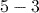• to indicate a negative number such as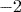.

Corresponding to these there are two different minus sign keys on the calculator:

•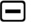, which is used for the operation of subtraction, as in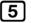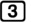•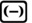, which is used for negative numbers, e.g.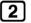.

In fact, some calculators permit thekey to be used for both purposes, but many other calculators require the equivalent of thekey to be used for negative numbers. For this reason we shall useto input negative numbers throughout this guide.

Note that if you attempt to usefor subtraction, for example, you will generate a Syntax Error.

## Activity 6 Subtraction and negative numbers

Calculate each of the following using your calculator. In each case, give your answer as a decimal not as a fraction.

1.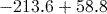2.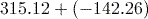3.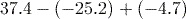4.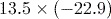5.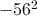### Answer

1.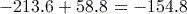Remember to press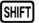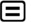to obtain a decimal answer.

2.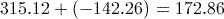3.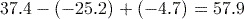4.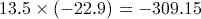5.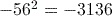You may have been surprised that the correct answer to part (5) is negative. According to the BIDMAS rules, the squaring is performed first, then the negative taken. If we wanted to calculate the square of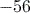, we write this mathematically as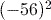and would need to use the brackets when evaluating it on a calculator.

MU123_1

### Take your learning further

Making the decision to study can be a big step, which is why you'll want a trusted University. The Open University has 50 years’ experience delivering flexible learning and 170,000 students are studying with us right now. Take a look at all Open University courses.

If you are new to University-level study, we offer two introductory routes to our qualifications. You could either choose to start with an Access module, or a module which allows you to count your previous learning towards an Open University qualification. Read our guide on Where to take your learning next for more information.

Not ready for formal University study? Then browse over 1000 free courses on OpenLearn and sign up to our newsletter to hear about new free courses as they are released.

Every year, thousands of students decide to study with The Open University. With over 120 qualifications, we’ve got the right course for you.

Request an Open University prospectus371